Next: Entropy and Quantum Mechanics Up: Statistical Thermodynamics Previous: Properties of Entropy

# Uses of Entropy

We have defined a new function called entropy, denoted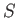, that parameterizes the amount of disorder in a macroscopic system. The entropy of an equilibrium macrostate is related to the number of accessible microstates,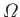, via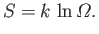(5.78)

On a macroscopic level, the increase in entropy due to a quasi-static change in which an infinitesimal amount of heat,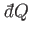, is absorbed by the system is given by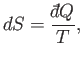(5.79)

whereis the absolute temperature of the system. The second law of thermodynamics states that the entropy of an isolated system can never spontaneously decrease. Let us now briefly examine some consequences of these results.

Consider two bodies,and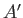, that are in thermal contact, but can do no work on one another. We know what is supposed to happen in this situation. Heat flows from the hotter to the colder of the two bodies until their temperatures become the same. Consider a quasi-static exchange of heat between the two bodies. According to the first law of thermodynamics, if an infinitesimal amount of heat,, is absorbed bythen infinitesimal heat,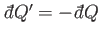, is absorbed by. The increase in the entropy of systemis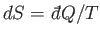, and the corresponding increase in the entropy ofis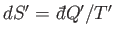. Here,and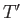are the temperatures of the two systems, respectively. Note thatis assumed to the sufficiently small that the heat transfer does not substantially modify the temperatures of either system. The change in entropy of the whole system is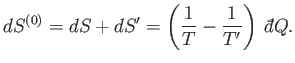(5.80)

This change must be positive or zero, according to the second law of thermodynamics, so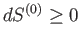. It follows thatis positive (i.e., heat flows fromto) when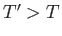, and vice versa. The spontaneous flow of heat only ceases when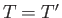. Thus, the direction of spontaneous heat flow is a consequence of the second law of thermodynamics. Note that the spontaneous flow of heat between bodies at different temperatures is always an irreversible process that increases the entropy, or disorder, of the universe.

Consider, now, the slightly more complicated situation in which the two systems can exchange heat, and also do work on one another via a movable partition. Suppose that the total volume is invariant, so that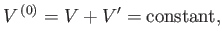(5.81)

whereand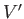are the volumes ofand, respectively. Consider a quasi-static change in which systemabsorbs an infinitesimal amount of heat,, and its volume simultaneously increases by an infinitesimal amount,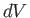. The infinitesimal amount of work done by systemis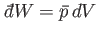(see Section 4.4), where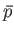is the mean pressure of. According to the first law of thermodynamics,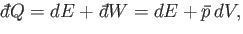(5.82)

where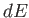is the change in the internal energy of. Because, the increase in entropy of systemis written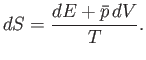(5.83)

Likewise, the increase in entropy of systemis given by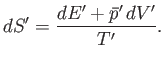(5.84)

According to Equation (5.83),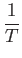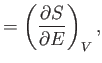(5.85)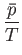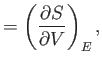(5.86)

where the subscripts are to remind us what is held constant in the partial derivatives. We can write an analogous pair of equations for the system.

The overall system is assumed to be isolated, so conservation of energy gives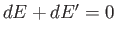. Furthermore, Equation (5.81) implies that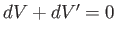. It follows that the total change in entropy is given by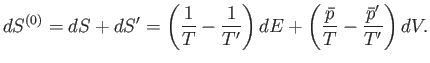(5.87)

The equilibrium state is the most probable state. (See Section 5.2.) According to statistical mechanics, this is equivalent to the state with the largest number of accessible microstates. Finally, Equation (5.78) implies that this is the maximum entropy state. The system can never spontaneously leave a maximum entropy state, because this would imply a spontaneous reduction in entropy, which is forbidden by the second law of thermodynamics. A maximum or minimum entropy state must satisfy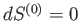for arbitrary small variations of the energy and external parameters. It follows from Equation (5.87) that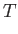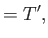(5.88)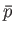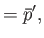(5.89)

for such a state. This corresponds to a maximum entropy state (i.e., an equilibrium state) provided that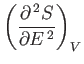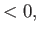(5.90)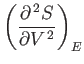(5.91)

with a similar pair of inequalities for system. The usual estimate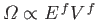(see Section 3.8), giving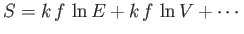, ensures that the previous inequalities are satisfied in conventional macroscopic systems. In the maximum entropy state, the systemsandhave equal temperatures (i.e., they are in thermal equilibrium), and equal pressures (i.e., they are in mechanical equilibrium). The second law of thermodynamics implies that the two interacting systems will evolve towards this state, and will then remain in it indefinitely (if left undisturbed).Next: Entropy and Quantum Mechanics Up: Statistical Thermodynamics Previous: Properties of Entropy
Richard Fitzpatrick 2016-01-25